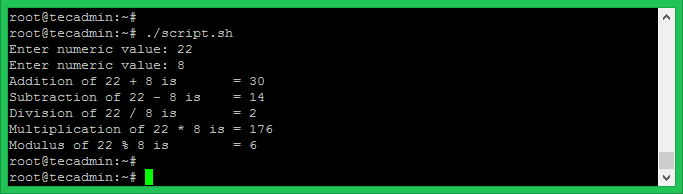1. Home
2. Bash
3. Bash Tutorial
4. Bash – Arithmetic Operations

# Bash Arithmetic Operations

You can perform arithmetic operations on numeric values in bash scripts. You can find many options to perform arithmetic operations on bash shell but below example will provide you simplest way.

Syntax:

``` ((expression))
((var1+var2))
((var1-var2))
((var1*var2))
((var1/var2))
```

## Bash Arithmetic Operations Example:

For example, take an input of two numeric values and perform all 5 basic arithmetic operations like below:## Increment and Decrement Operator:

Bash also used incremnt (++) and decrement (–) operators. Both uses in two types pre-increment/post-increment and pre-decrement/post-decrement.

Similarly, try pre-decrement and post-decrement operators in the bash shell.

Tags